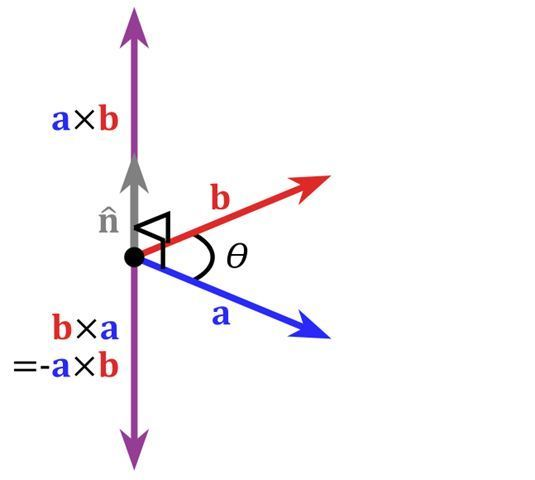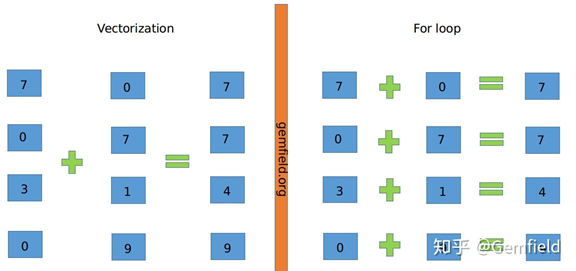• 矢量操作可以使用 ogr这类基础方法进行，但同时还有一些高级库支持更便捷的矢量操作，例如Fiona(shapely)、pyshp(shapefile)、geopandas等。教程资料导航ogr教程资料：shapely教程资料：pyshp教程资料：GeoPandas...

矢量操作可以使用 ogr这类基础方法进行，但同时还有一些高级库支持更便捷的矢量操作，例如Fiona(shapely)、pyshp(shapefile)、geopandas等。

教程资料导航

ogr教程资料：

shapely教程资料：

pyshp教程资料：

GeoPandas教程资料：

Fiona教程资料：

以下为判断点在面内的示例：

Solution 1 with

Fiona

import fiona

from shapely.geometry import shape,mapping, Point, Polygon, MultiPolygon

multipol = fiona.open("multipol.shp")

multi= multipol.next() # only one feature in the shapefile

print multi

{'geometry': {'type': 'MultiPolygon', 'coordinates': [[[(-0.5275288092189501, 0.5569782330345711), (-0.11779769526248396, 0.29065300896286816), (-0.25608194622279135, 0.01920614596670933), (-0.709346991037132, -0.08834827144686286), (-0.8629961587708066, 0.18309859154929575), (-0.734955185659411, 0.39820742637644047), (-0.5275288092189501, 0.5569782330345711)]], [[(0.19974391805377723, 0.060179257362355965), (0.5480153649167734, 0.1293213828425096), (0.729833546734955, 0.03969270166453265), (0.8143405889884763, -0.13956466069142115), (0.701664532650448, -0.38540332906530095), (0.4763124199743918, -0.5006402048655569), (0.26888604353393086, -0.4238156209987196), (0.18950064020486557, -0.2291933418693981), (0.19974391805377723, 0.060179257362355965)]], [[(-0.3764404609475033, -0.295774647887324), (-0.11523687580025621, -0.3597951344430217), (-0.033290653008962945, -0.5800256081946222), (-0.11523687580025621, -0.7413572343149808), (-0.3072983354673495, -0.8591549295774648), (-0.58898847631242, -0.6927016645326505), (-0.6555697823303457, -0.4750320102432779), (-0.3764404609475033, -0.295774647887324)]]]}, 'type': 'Feature', 'id': '0', 'properties': OrderedDict([(u'id', 1)])}

Fiona interprets the feature as a MultiPolygon and you can apply the solution presented in

More Efficient Spatial join in Python without QGIS, ArcGIS, PostGIS, etc (1)

points= ([pt for pt in fiona.open("points.shp")])

for i, pt in enumerate(points):

point = shape(pt['geometry'])

if point.within(shape(multi['geometry'])):

print i, shape(points[i]['geometry'])

1 POINT (-0.58898847631242 0.17797695262484)

3 POINT (0.4993597951344431 -0.06017925736235585)

5 POINT (-0.3764404609475033 -0.4750320102432779)

6 POINT (-0.3098591549295775 -0.6312419974391805)

Solution 2 with

pyshp (shapefile)

and the

geo_interface

(GeoJSON like) protocol

import shapefile

points = [pt.shape.__geo_interface__ for pt in pts.shapeRecords()]

multi = shape(polys.shapeRecords().shape.__geo_interface__) # 1 polygon

print multi

MULTIPOLYGON (((-0.5275288092189501 0.5569782330345711, -0.117797695262484 0.2906530089628682, -0.2560819462227913 0.01920614596670933, -0.7093469910371319 -0.08834827144686286, -0.8629961587708066 0.1830985915492958, -0.734955185659411 0.3982074263764405, -0.5275288092189501 0.5569782330345711)), ((0.1997439180537772 0.06017925736235596, 0.5480153649167734 0.1293213828425096, 0.729833546734955 0.03969270166453265, 0.8143405889884763 -0.1395646606914211, 0.701664532650448 -0.3854033290653009, 0.4763124199743918 -0.5006402048655569, 0.2688860435339309 -0.4238156209987196, 0.1895006402048656 -0.2291933418693981, 0.1997439180537772 0.06017925736235596)), ((-0.3764404609475033 -0.295774647887324, -0.1152368758002562 -0.3597951344430217, -0.03329065300896294 -0.5800256081946222, -0.1152368758002562 -0.7413572343149808, -0.3072983354673495 -0.8591549295774648, -0.58898847631242 -0.6927016645326505, -0.6555697823303457 -0.4750320102432779, -0.3764404609475033 -0.295774647887324)))

for i, pt in enumerate(points):

point = shape(pt)

if point.within(multi):

print i, shape(points[i])

1 POINT (-0.58898847631242 0.17797695262484)

3 POINT (0.4993597951344431 -0.06017925736235585)

5 POINT (-0.3764404609475033 -0.4750320102432779)

6 POINT (-0.3098591549295775 -0.6312419974391805)

Solution 3 with ogr and the

geo_interface

protocol (

Python Geo_interface applications

)

from osgeo import ogr

import json

def records(file):

# generator

for i in range(layer.GetFeatureCount()):

feature = layer.GetFeature(i)

points = [pt for pt in records("point_multi_contains.shp")]

multipol = records("multipol.shp")

multi = multipol.next() # 1 feature

for i, pt in enumerate(points):

point = shape(pt['geometry'])

if point.within(shape(multi['geometry'])):

print i, shape(points[i]['geometry'])

1 POINT (-0.58898847631242 0.17797695262484)

3 POINT (0.499359795134443 -0.060179257362356)

5 POINT (-0.376440460947503 -0.475032010243278)

6 POINT (-0.309859154929577 -0.631241997439181)

import geopandas

point = geopandas.GeoDataFrame.from_file('points.shp')

poly = geopandas.GeoDataFrame.from_file('multipol.shp')

from geopandas.tools import sjoin

pointInPolys = sjoin(point, poly, how='left')

grouped = pointInPolys.groupby('index_right')

list(grouped)

[(0.0, geometry id_left index_right id_right

1 POINT (-0.58898847631242 0.17797695262484) None 0.0 1.0

3 POINT (0.4993597951344431 -0.06017925736235585) None 0.0 1.0

5 POINT (-0.3764404609475033 -0.4750320102432779) None 0.0 1.0

6 POINT (-0.3098591549295775 -0.6312419974391805) None 0.0 1.0 ]

print grouped.groups

{0.0: [1, 3, 5, 6]}

The points 1,3,5,6 falls within the boundaries of the MultiPolygon

展开全文• 2、几何表示：向量可以用向线段来表示.向线段的长度表示向量的大小,箭头所指的方向表示向量的方向。(若规定线段AB的端点A为起点,B为终点,则线段就具有了从起点A到终点B的方向和长度.这种具有方向和长度的线段...

1、代数表示：一般印刷用黑体小写字母α、β、γ … 或a、b、c … 等来表示,手写用在a、b、c…等字母上加一箭头表示。

2、几何表示：向量可以用有向线段来表示.有向线段的长度表示向量的大小,箭头所指的方向表示向量的方向。

(若规定线段AB的端点A为起点,B为终点,则线段就具有了从起点A到终点B的方向和长度.这种具有方向和长度的线段叫做有向线段.)

3、坐标表示：

(1)在平面直角坐标系中,分别取与x轴、y轴方向相同的两个单位向量i,j作为一组基底.a为平面直角坐标系内的任意向量,以坐标原点O为起点作向量OP=a。

由平面向量基本定理知,有且只有一对实数(x,y),使得 a=向量OP=xi+yj,因此把实数对(x,y)叫做向量a的坐标,记作a=(x,y).这就是向量a的坐标表示.其中(x,y)就是点P的坐标.向量OP称为点P的位置向量。

(2) 在立体三维坐标系中,分别取与x轴、y轴,z轴方向相同的3个单位向量i,j,k作为一组基底.若a为该坐标系内的任意向量,以坐标原点O为起点作向量OP=a。

由空间基本定理知,有且只有一组实数(x,y,z),使得 a=向量OP=xi+yj+zk,因此把实数对(x,y,k)叫做向量a的坐标,记作a=(x,y,z).这就是向量a的坐标表示.其中(x,y,k),也就是点P的坐标.向量OP称为点P的位置向量。

(3) 当然,对于空间多维向量,可以通过类推得到 。注：

向量的定义：

在数学中，向量(也称为欧几里得向量、几何向量、矢量)，指具有大小(magnitude)和方向的量。它可以形象化地表示为带箭头的线段。箭头所指：代表向量的方向；线段长度：代表向量的大小。与向量对应的只有大小，没有方向的量叫做数量(物理学中称标量)。

向量的记法：印刷体记作粗体的字母(如a、b、u、v)，书写时在字母顶上加一小箭头“→”。 如果给定向量的起点(A)和终点(B)，可将向量记作AB(并于顶上加→)。在空间直角坐标系中，也能把向量以数对形式表示，例如Oxy平面中(2,3)是一向量。

在物理学和工程学中，几何向量更常被称为矢量。许多物理量都是矢量，比如一个物体的位移，球撞向墙而对其施加的力等等。与之相对的是标量，即只有大小而没有方向的量。一些与向量有关的定义亦与物理概念有密切的联系，例如向量势对应于物理中的势能。

几何向量的概念在线性代数中经由抽象化，得到更一般的向量概念。此处向量定义为向量空间的元素，要注意这些抽象意义上的向量不一定以数对表示，大小和方向的概念亦不一定适用。因此，平日阅读时需按照语境来区分文中所说的"向量"是哪一种概念。

不过，依然可以找出一个向量空间的基来设置坐标系，也可以透过选取恰当的定义，在向量空间上介定范数和内积，这允许我们把抽象意义上的向量类比为具体的几何向量。

扩展资料：

向量的运算法则：(向量的加法满足平行四边形法则和三角形法则)

1、向量的加法

OB+OA=OC.

a+b=(x+x',y+y').

a+0=0+a=a.

向量加法的运算律：

交换律：a+b=b+a；

结合律：(a+b)+c=a+(b+c).

2、向量的减法

如果a、b是互为相反的向量,那么a=-b,b=-a,a+b=0.0的反向量为0

AB-AC=CB.

a=(x,y)b=(x',y') 则a-b=(x-x',y-y').

3、数乘向量

实数λ和向量a的乘积是一个向量,记作λa,且∣λa∣=∣λ∣·∣a∣.

当λ＞0时,λa与a同方向；

向量的数乘法则：

当λ＜0时,λa与a反方向；

向量的数乘当λ=0时,λa=0,方向任意.

当a=0时,对于任意实数λ,都有λa=0.

注：按定义知,如果λa=0,那么λ=0或a=0.

实数λ叫做向量a的系数,乘数向量λa的几何意义就是将表示向量a的有向线段伸长或压缩.

当∣λ∣＞1时,表示向量a的有向线段在原方向(λ＞0)或反方向(λ＜0)上伸长为原来的∣λ∣倍；

当∣λ∣＜1时,表示向量a的有向线段在原方向(λ＞0)或××反方向(λ＜0)上缩短为原来的∣λ∣倍.

注：数与向量的乘法满足下面的运算律 :

①结合律：(λa)·b=λ(a·b)=(a·λb).

②向量对于数的分配律(第一分配律)：(λ+μ)a=λa+μa.

③数对于向量的分配律(第二分配律)：λ(a+b)=λa+λb.

④数乘向量的消去律：① 如果实数λ≠0且λa=λb,那么a=b.② 如果a≠0且λa=μa,那么λ=μ.

4、向量的数量积

定义：已知两个非零向量a,b.作OA=a,OB=b,则角AOB称作向量a和向量b的夹角,记作〈a,b〉并规定0≤〈a,b〉≤π

定义：两个向量的数量积(内积、点积)是一个数量,记作a·b.若a、b不共线,则a·b=|a|·|b|·cos〈a,b〉；若a、b共线,则a·b=+-∣a∣∣b∣.

向量的数量积的坐标表示：a·b=x·x'+y·y'.

向量的数量积的运算律 ：

①a·b=b·a(交换律)；

②(λa)·b=λ(a·b)(关于数乘法的结合律)；

③(a+b)·c=a·c+b·c(分配律)；

向量的数量积的性质 ：

a·a=|a|的平方.

a⊥b 〈=〉a·b=0.

|a·b|≤|a|·|b|.(该公式证明如下：|a·b|=|a|·|b|·|cosα| 因为0≤|cosα|≤1,所以|a·b|≤|a|·|b|)

注：向量的数量积与实数运算的主要不同点 ：

①向量的数量积不满足结合律,即：(a·b)·c≠a·(b·c)；例如：(a·b)^2≠a^2·b^2.

②向量的数量积不满足消去律,即：由 a·b=a·c (a≠0),推不出 b=c.

③|a·b|≠|a|·|b|

④由 |a|=|b| ,推不出 a=b或a=-b.

⑤向量的向量积

定义：两个向量a和b的向量积(外积、叉积)是一个向量,记作a×b(这里并不是乘号,只是一种表示方法,与“·”不同,也可记做“∧”)。

若a、b不共线,则a×b的模是：∣a×b∣=|a|·|b|·sin〈a,b〉；a×b的方向是：垂直于a和b,且a、b和a×b按这个次序构成右手系.若a、b共线,则a×b=0。

向量的向量积性质：

∣a×b∣是以a和b为边的平行四边形面积.

a×a=0.

a垂直b〈=〉a×b=|a||b|.

向量的向量积运算律 ：

a×b=-b×a；

(λa)×b=λ(a×b)=a×(λb)；

a×(b+c)=a×b+a×c.

注：向量没有除法,“向量AB/向量CD”是没有意义的。

参考资料：百度百科-向量

展开全文c语言中向量表示方法
• MATLAB入门教程* 1* 下一篇文章1．MATLAB的基本知识1-1、基本运算与函数在MATLAB下进行基本数学运算，只需将运算式直接打入提示号(>>)之後，并按入Enter键即可。例如：>> (5*2+1.3-0.8)*10/25ans =4....MATLAB入门教程

* 1

* 下一篇文章

1．MATLAB的基本知识

1-1、基本运算与函数

在MATLAB下进行基本数学运算，只需将运算式直接打入提示号(>>)之後，并按入Enter键即可。例如：

>> (5*2+1.3-0.8)*10/25

ans =4.2000

小提示： ">>"是MATLAB的提示符号(Prompt)，但在PC中文视窗系统下，由於编码方式不同，此提示符号常会消失不见，但这并不会影响到MATLAB的运算结果。

我们也可将上述运算式的结果设定给另一个变数x：

x = (5*2+1.3-0.8)*10^2/25

x = 42

此时MATLAB会直接显示x的值。由上例可知，MATLAB认识所有一般常用到的加(+)、减(-)、乘(*)、除(/)的数学运算符号，以及幂次运算(^)。

小提示： MATLAB将所有变数均存成double的形式，所以不需经过变数宣告(Variable declaration)。MATLAB同时也会自动进行记忆体的使用和回收，而不必像C语言,必须由使用者一一指定.这些功能使的MATLAB易学易用，使用者可专心致力於撰写程式，而不必被软体枝节问题所干扰。

若不想让MATLAB每次都显示运算结果，只需在运算式最後加上分号(；)即可，如下例：

y = sin(10)*exp(-0.3*4^2);

若要显示变数y的值，直接键入y即可：

>>y

y =-0.0045

在上例中，sin是正弦函数，exp是指数函数，这些都是MATLAB常用到的数学函数。

下表即为MATLAB常用的基本数学函数及三角函数：

小整理：MATLAB常用的基本数学函数

abs(x)：纯量的绝对值或向量的长度

angle(z)：复 数z的相角(Phase angle)

sqrt(x)：开平方

real(z)：复数z的实部

imag(z)：复数z的虚 部

conj(z)：复数z的共轭复数

round(x)：四舍五入至最近整数

fix(x)：无论正负，舍去小数至最近整数

floor(x)：地板函数，即舍去正小数至最近整数

ceil(x)：天花板函数，即加入正小数至最近整数

rat(x)：将实数x化为分数表示

rats(x)：将实数x化为多项分数展开

sign(x)：符号函数 (Signum function)。

当x<0时，sign(x)=-1；

当x=0时，sign(x)=0;

当x>0时，sign(x)=1。

> 小整理：MATLAB常用的三角函数

sin(x)：正弦函数

cos(x)：馀弦函数

tan(x)：正切函数

asin(x)：反正弦函数

acos(x)：反馀弦函数

atan(x)：反正切函数

atan2(x,y)：四象限的反正切函数

sinh(x)：超越正弦函数

cosh(x)：超越馀弦函数

tanh(x)：超越正切函数

asinh(x)：反超越正弦函数

acosh(x)：反超越馀弦函数

atanh(x)：反超越正切函数

变数也可用来存放向量或矩阵，并进行各种运算，如下例的列向量(Row vector)运算：

x = [1 3 5 2];

y = 2*x+1

y = 3 7 11 5

小提示：变数命名的规则

1.第一个字母必须是英文字母 2.字母间不可留空格 3.最多只能有19个字母，MATLAB会忽略多馀字母

我们可以随意更改、增加或删除向量的元素：

y(3) = 2 % 更改第三个元素

y =3 7 2 5

y(6) = 10 % 加入第六个元素

y = 3 7 2 5 0 10

y(4) = [] % 删除第四个元素，

y = 3 7 2 0 10

x(2)*3+y(4) % 取出x的第二个元素和y的第四个元素来做运算

ans = 9

y(2:4)-1 % 取出y的第二至第四个元素来做运算

ans = 6 1 -1

在上例中，2:4代表一个由2、3、4组成的向量

若对MATLAB函数用法有疑问，可随时使用help来寻求线上支援(on-line help)：help linspace

小整理：MATLAB的查询命令

help：用来查询已知命令的用法。例如已知inv是用来计算反矩阵，键入help inv即可得知有关inv命令的用法。(键入help help则显示help的用法，请试看看！) lookfor：用来寻找未知的命令。例如要寻找计算反矩阵的命令，可键入 lookfor inverse，MATLAB即会列出所有和关键字inverse相关的指令。找到所需的命令後 ，即可用help进一步找出其用法。(lookfor事实上是对所有在搜寻路径下的M档案进行关键字对第一注解行的比对，详见後叙。)

将列向量转置(Transpose)後，即可得到行向量(Column vector)：

z = x'

z = 4.0000

5.2000

6.4000

7.6000

8.8000

10.0000

不论是行向量或列向量，我们均可用相同的函数找出其元素个数、最大值、最小值等：

length(z) % z的元素个数

ans = 6

max(z) % z的最大值

ans = 10

min(z) % z的最小值

ans = 4

小整理：适用於向量的常用函数有：

min(x): 向量x的元素的最小值

max(x): 向量x的元素的最大值

mean(x): 向量x的元素的平均值

median(x): 向量x的元素的中位数

std(x): 向量x的元素的标准差

diff(x): 向量x的相邻元素的差

sort(x): 对向量x的元素进行排序(Sorting)

length(x): 向量x的元素个数

norm(x): 向量x的欧氏(Euclidean)长度

sum(x): 向量x的元素总和

prod(x): 向量x的元素总乘积

cumsum(x): 向量x的累计元素总和

cumprod(x): 向量x的累计元素总乘积

dot(x, y): 向量x和y的内 积

cross(x, y): 向量x和y的外积 (大部份的向量函数也可适用於矩阵，详见下述。)

若要输入矩阵，则必须在每一列结尾加上分号(；)，如下例：

A = [1 2 3 4; 5 6 7 8; 9 10 11 12];

A =

1 2 3 4

5 6 7 8

9 10 11 12

同样地，我们可以对矩阵进行各种处理：

A(2,3) = 5 % 改变位於第二列，第三行的元素值

A =

1 2 3 4

5 6 5 8

9 10 11 12

B = A(2,1:3) % 取出部份矩阵B

B = 5 6 5

A = [A B'] % 将B转置後以行向量并入A

A =

1 2 3 4 5

5 6 5 8 6

9 10 11 12 5

A(:, 2) = [] % 删除第二行(：代表所有列)

A =

1 3 4 5

5 5 8 6

9 11 12 5

A = [A; 4 3 2 1] % 加入第四列

A =

1 3 4 5

5 5 8 6

9 11 12 5

4 3 2 1

A([1 4], :) = [] % 删除第一和第四列(：代表所有行)

A =

5 5 8 6

9 11 12 5

这几种矩阵处理的方式可以相互叠代运用，产生各种意想不到的效果，就看各位的巧思和创意。

小提示：在MATLAB的内部资料结构中,每一个矩阵都是一个以行为主(Column-oriented )的阵列(Array)因此对於矩阵元素的存取，我们可用一维或二维的索引(Index)来定址。举例来说，在上述矩阵A中，位於第二列、第三行的元素可写为A(2,3) (二维索引)或A(6)(一维索引，即将所有直行进行堆叠後的第六个元素)。

此外，若要重新安排矩阵的形状，可用reshape命令：

B = reshape(A, 4, 2) % 4是新矩阵的列数，2是新矩阵的行数

B =

5 8

9 12

5 6

11 5

小提示： A(:)就是将矩阵A每一列堆叠起来，成为一个行向量，而这也是MATLAB变数的内部储存方式。以前例而言，reshape(A, 8, 1)和A(:)同样都会产生一个8x1的矩阵。

MATLAB可在同时执行数个命令，只要以逗号或分号将命令隔开：

x = sin(pi/3); y = x^2; z = y*10,

z =

7.5000

若一个数学运算是太长，可用三个句点将其延伸到下一行：

z = 10*sin(pi/3)* ...

sin(pi/3);

若要检视现存於工作空间(Workspace)的变数，可键入who：

who

testfile x

这些是由使用者定义的变数。若要知道这些变

◆◆

评论读取中....

请登录后再发表评论!

◆◆

修改失败，请稍后尝试

展开全文• 向量化运算

2021-09-15 15:02:45
向量运算OLAP Clickhouse、doris、spark(2.x以后)、 hive（0.13.0以后）、presto SIMD SIMD全称Single Instruction Multiple Data，单指令多数据流，能够复制多个操作数，并把它们打包在大型寄存器的一组指令集。 ...

向量化运算OLAP

Clickhouse、dorisDB(starrocks)、spark(2.x以后)、 hive（0.13.0以后）、presto

SIMD

SIMD全称Single Instruction Multiple Data，单指令多数据流，能够复制多个操作数，并把它们打包在大型寄存器的一组指令集。
前提需要支持SIMD的CPU才能发挥其特性。

性能上优势

以加法指令为例，单指令单数据（SISD）的CPU对加法指令译码后，执行部件先访问内存，取得第一个操作数；之后再一次访问内存，取得第二个操作数；随后才能进行求和运算。而在SIMD型的CPU中，指令译码后几个执行部件同时访问内存，一次性获得所有操作数进行运算。这个特点使SIMD特别适合于多媒体应用等数据密集型运算。

注： 上面的内存指的是CPU寄存器

什么是vectorization

​ 向量化计算(vectorization)，向量化计算是一种特殊的并行计算的方式，它可以在同一时间执行多次操作，通常是对不同的数据执行同样的一个或一批指令，或者说把指令应用于一个数组/向量​ 上图中，左侧为vectorization，右侧为寻常的For loop计算。将多次for循环计算变成一次计算完全仰仗于CPU的SIMD指令集，SIMD指令可以在一条cpu指令上处理2、4、8或者更多份的数据。

​ 因此简单来说，向量化计算就是将一个loop处理一个array的时候每次处理1个数据共处理N次，转化为vectorization处理一个array的时候每次同时处理8个数据共处理N/8次。

向量化运算的实例

​ 在Python的numpy库中使用向量化(Vectorization)计算，速度是非向量化(non-Vectorization)计算（即循环）的700倍（当前开发环境），因为向量化计算使用了python的内建函数，调用了CPU/GPU的SIMD指令集进行计算，大大减少了因为python高级语言执行损耗的时间。

实例:

import numpy as np
import time

cnt=10000000
a = np.random.rand(cnt)
b = np.random.rand(cnt)

tic = time.time()
c = np.dot(a, b)
toc = time.time()
print("c: %f" % c)
print("vectorized version:" + str(1000*(toc-tic)) + "ms")

c = 0
tic = time.time()
for i in range(cnt):
c += a[i] * b[i]
toc = time.time()
print("c: %f" % c)
print("for loop:" + str(1000*(toc-tic)) + "ms")

结果;

c: 2499089.213332
vectorized version:5.998373031616211ms
c: 2499089.213332
for loop:4685.6348514556885ms

展开全文python 人工智能
• 向量运算表示一定程度的并行度，该并行度允许在单个CPU周期中执行更多工作，因此可以显着提高性能。例如，给定两个向量，每个向量覆盖八个整数（八个通道）的序列，那么可以使用单个硬件指令将这两个向量相加。向量...
• 向量 标量积（内积） 标量积（又称点乘、点积、数量积）：两个向量相乘得到一个标量。 几何意义： 代数定义： 向量积（外积） 向量积（又称叉乘、叉积、矢量积）：两个向量相乘得到一个向量向量积定义...线性代数 数学 矩阵
• 本文含有：向量的创建与运算两个方便创建向量的函数：rep()与seq()如何访问向量中的元素首先先明白个要点：R区分大小写，向量A和向量a是两个不同的向量；R可以识别负数，如5+-2可以输出结果3R是计数时是1-base，也...
• 本文介绍了向量的定义、向量的模、负向量、单位向量、零向量以及向量加减法的三实现方法。人工智能 数学 线性代数 向量
• 在WebGL的实际应用中我们广泛使用向量的几何运算来计算角度、距离，判断点线、点面之间的关系，比如物体之间的碰撞检测。本文简要介绍三维计算机图形学中常用的基本运算的概念及其用途。点积(Dot Product)点乘比较...
• R 语言里所有的东西都被称为对象，R语言中常见的数据类型几下几种，分别是字符型 (character)、数值型 (numeric)、复数型 (complex)以及逻辑型 (logical)。通过mode()函数可以查看一个对象的类型。 R语言中的基本...r语言 开发语言
• 这个问题不同的方面，我可以给你一些提示，告诉你如何解决这些问题。请注意，这些都是建议，你肯定需要看看你最喜欢哪一个。支持线性代数您提到要支持线性代数，例如向量加法(元素加法)、叉积和内积。这些可用于...
• （计算海明距离的一方法，就是对两个位串进行异或（xor）运算，并计算出异或运算结果中1的个数。例如110和011这两个位串，对它们进行异或运算，其结果是 110⊕011=101 异或结果中含有两个1，因此110和011之间的...数据分析
• 索引一、什么是范式二、向量范式1-范数2-范数P-范数∞-范数-∞-范数三、矩阵范式1-范数2-范数∞-范数F-范数四、小结 一、什么是范式 范数，是具有“长度”概念的函数。在线性代数、泛函分析及相关的数学领域，范数...矩阵 泛函分析 线性代数
• 目录 一、Gradient descent for liner regression - 线性回归: 1.线性回归的梯度下降法： ...向量运算： 1.Addition and scalar multiplication - 加法和标量乘法: 2.Matrix - Vector multiplicat.机器学习 深度学习 人工智能
• ClickHouse在计算层做了非常细致的工作，竭尽所能榨干硬件能力，提升查询速度。它实现了单机多核并行、分布式计算、向量化执行与SIMD指令、代码生成等多种重要技术。多核并行ClickH...大数据 java 编程语言 数据库 spark
• 计算机系统的位运算与逻辑运算一、位1、定义二进制数字系统中数据存储的最小单位，即每个二进制数0或1就称为位。位也叫比特(bit)，8个bit组成一个字节(byte)，每个字节表示程序中的某些文本字符。字长(word size)...
• 向量用于使用一个名称对相关信号进行分组，以使其更易于操作。例如，电线[7：0] w; 声明一个名为w的8位向量，该向量在功能上等同于具有8条独立的导线。 请注意，向量的声明将维放置在向量名称之前，这与C语法相比并...
• 诚然，没有一门语言能够撼动matlab的矩阵或科学计算在学术圈的地位，因其简洁的语法(matrix是其基本数据类型)，因其矩阵运算的便捷，因其术业专攻(matlab：为科学计算而生)，因其名字matlab：matrix laboratory，...
• 前面介绍的进行向量运算的apply族函数来自基础包base，而本文介绍的map族函数则来自purrr包，是tidyverse家族的一员。与apply相比，map函数在逻辑上更易于理解，并...python js 数据结构 java html
• 本发明属于机器学习领域，特别涉及一支持向量机的最佳参数组合(C，σ)快速搜索方法。背景技术：支持向量机是一二类分类模型，其基本模型是定义在特征空间上的间隔最大的线性分类器。Cortes和Vapnik首次提出线性...支持向量机c语言代码
• 3.10 矩阵运算在几何光学中的应用3.9几种典型系统的理想光学系统性质1.望远镜系统2.显微镜系统3.照相物镜系统3.10 矩阵运算在几何光学中的应用（了解）1.近轴光的矩阵表示2.物像矩阵3.用高斯常数表示系统的基点位置...个人开发
• MATLAB向量

2021-04-19 07:32:18
向量向量MATLAB 行向量：创建行向量括在方括号中的元素的集合，用空格或逗号分隔的元素。r = [7 8 9 10 11]执行上述语句，返回下述结果：r =Columns 1 through 47 8 9 10Column 511MATLAB 列向量：创建列向量括...
• 其实多维数组的索引还是很好理解的，例如下例中，我们可以看到对于一个张量，也就是b，对其索引是，[i,j,k]中的i表示选择第个二维数组， 然后j表示取二维数组中的第个行向量，k表示取行向量中的第个元素。...
• 从初到末的箭头(物理角度，表示一运动过程)有序的数字列表(计算机/数学角度)[1,2]加和数乘运算有意义的anything(抽象意义)12两理解之间的关系就是线性代数的奥秘，即几何角度与数值角度。一个向量的坐标由一对数...向量叉乘矩阵表示
• matlab中如何定义向量

千次阅读 2021-04-18 05:22:17
Matlab中生成向量的三方法在Matlab中，如何才能生成向量，生成向量的方法又多少？相信这是每一个初学者都想知道的问题。这里小编将向大家详细介绍Matlab中生成向量的三方法。方法一：直接输入法1这是最简单...
• 单位向量就是长度为1的向量、标量：标量是一个只有数值大小 没有方向，部分征服之分。通俗来说标量只有大小没有方向的量。向量和标量的区别就是 向量方向的。标量是没有方向的。向量的点乘叉乘：点乘又叫向量...向量叉乘矩阵表示
• 其中a·b=｜a｜·｜b｜cosθ,｜a｜、｜b｜是两向量的模,θ是两向量之间的夹角(0≤θ≤π).以上a与b均为向量，点乘，也叫向量的内积、数量积。 几何意义：一个向量长度和它在另一个向量投影长度的乘积。 叉乘、向量积...
• 向量检索是大数据应用很关键的一个环节，在多种推荐，搜索中都广泛的应用。本文分类介绍业界向量检索算法的原理，让读者对向量检索一个初步的认识。聚类 大数据 信息检索 算法
• MATLAB矩阵及其运算

千次阅读 2021-04-18 05:09:55
第2章MATLAB矩阵及其运算2.1变量和数据操作2.2MATLAB矩阵2.3MATLAB运算2.4矩阵分析2.5矩阵的超越函数2.6字符串2.7结构数据和单元数据2.8稀疏矩阵2.1变量和数据操作2.1.1变量与赋值1．变量命名在MATLAB 6.5中，变量名......